﻿ Inventory Optimization for Combined Stock-depended and Stochastic DemandPublications are Open
Access in this journal
Article Versions
Export Article
• Normal Style
• MLA Style
• APA Style
• Chicago Style
Research Article
Open Access Peer-reviewed

### Inventory Optimization for Combined Stock-depended and Stochastic Demand

Nasib Al Habib , Shuva Ghosh
American Journal of Industrial Engineering. 2018, 5(1), 1-11. DOI: 10.12691/ajie-5-1-1
Published online: February 06, 2018

### Abstract

This work develops an optimal inventory policy for stock-dependent demand items, taking into account both lead time and lead time demand uncertainties. (Q,R) modeling approach has been implemented to deal with uncertainties of demand and lead time. Unlike previous models, this model combines a deterministic approach with a stochastic approach to formulate a hybrid one. Holding cost per unit per unit time is considered an increasing function of quantity in storage up to reorder point and lead time demand is assumed to be proportional to the span of lead time. Lead time is normally distributed and since demand during lead time is proportional to it, the lead time demand also follows a normal distribution. This is a single objective unconstrained optimization model that aims at minimizing inventory cost where the decision variables are order quantity and reorder point.

### 1. Introduction

Inventory or stocks are the goods and materials that a stores for the purpose of resale (or repair) and managing supply chain uncertainties. Inventory exists in the supply chain because of a mismatch between supply and demand. This mismatch is intentional at a steel manufacturer, where it is economical to manufacture in large lots that are then stored for future sales. The mismatch is also intentional at a retail store where inventory is held in anticipation of future demand. An important role that inventory plays in the supply chain is to increase the amount of demand that can be satisfied by having the product ready and available when the customer wants it. Another significant role that inventory plays is to reduce cost by exploiting economies of scale that may exist during production and distribution. This article is concerned about inventory optimization of stock-depended items, for example, fashionable goods or food items. To measure the performance of replenishment policies of inventory, service-level is normally used in inventory management. In this case, an optimal solution model is found so that an optimal size of backorders can be derived. On the other hand, fill-rate which is related to inventory management stands for the measurement of customer satisfaction. It measures the amount of demand that was satisfied from the on hand inventory.

Dave et al. 1 were the first to study a deteriorating inventory with linearly increasing demand when shortages are not allowed. The rate of deterioration was assumed constant in their work. In this field, some of the recent work has been done by Chung et al. 2, where the deterioration is time dependent. Wee 3 also developed an inventory model with deteriorating items where deterioration follows Weibull distribution. Chang et al. 4 studied an inventory model with time-varying demand and partial backlogging. The demand was a linear function of time in their research. Goyal et al. 5 developed the recent trends of modeling in deteriorating item inventory. They classified inventory models based on demand variations and various other conditions or constraints. Ouyang et al. 6 formulated an inventory model for deteriorating items with exponential declining demand and partial backlogging. The effects of learning and forgetting on the optimal production lot size for deteriorating items with time-varying demand and deterioration rates were studied by Alamri et al. 7. Dye et al. 8 formulated an optimal selling price and lot size with a varying rate of deterioration and exponential partial backlogging.

Liao 9 gave an economic order quantity (EOQ) model with non-instantaneous receipt and exponential deteriorating item under two level trade credits. Lee et al. 10 developed a note on EOQ model for items with mixtures of exponentially distributed deterioration, shortages and time varying demand. Huang et al. 11 presented a simple algebraic approach to find the exact optimal lead time and the optimal cycle time in steady market demand situations where both lead time and demand were deterministic. Pareek et al. 12 developed a deterministic inventory model for deteriorating items with resale value and shortages. To allow shortage is a more practical approach to inventory optimization as shortage might always occur in real situations. Skouri et al. 13 developed an inventory model with ramp-type demand rate, partial backlogging, and Weibull's deterioration rate. Mishra et al. 14 developed a deteriorating inventory model for waiting time partial backlogging when demand and deterioration rate is constant. Mandal 15 developed an EOQ inventory model for Weibull-distributed deteriorating items under ramp-type demand and shortages. Mishra et al. 16 developed an inventory model for deteriorating items with time-dependent demand and time-varying holding cost under partial backlogging. Inventory model for deteriorating items with predetermined life under quadratic demand and nonlinear holding cost has been developed by Niketa D. Trivedi et al 17.

The pioneer researchers who formulated inventory models taking initial stock-level dependent demand are Gupta et al. 18. Mandal et al. 19 corrected the flaw in Gupta et al. model using profit maximization rather than cost minimization as the objective. They proposed an inventory model in which shortages are allowed and demand is dependent on stock-level. Baker et al. 20 developed an inventory model taking demand rate in polynomial functional form dependent on inventory level. The same functional form was used by Datta et al. 21, who proposed an inventory model for deteriorating items, inventory-level dependent demand and shortages. In this model the rate of deterioration is assumed to be variable. Sarker et al. 22 took demand to be dependent on inventory level incorporating an entirely new concept of decrease in demand (due to ageing of inventory or products reaching closer to their expiry date). Inventory models with stock-dependent demand and nonlinear holding costs for deteriorating items was developed by Chun-Tao Chang 23. An inventory model with both stock-dependent demand rate and stock-dependent holding cost rate was developed by 24. A partial backlogging inventory model for non-instantaneous deteriorating items with special stock-dependent demand was proposed by et al. 25. M. Maragatham et al. 26 proposed an inventory model for deteriorating items with time dependent holding cost.

Montgomery et al. 27 developed both deterministic and stochastic models considering the situation in which a fraction of demand during the stock out period is backordered and remaining is lost forever. Rosenberg 28 developed a lot-size inventory model with partial backlogging taking “fictitious demand rate” that simplifies the analysis. Padmanabhan et al. 29 proposed an inventory model for perishable items taking constant rate of deterioration, incorporating the three cases of complete, partial and no backlogging. Zeng 30 developed an inventory model using partial backordering approach and minimizing the total cost function. This model identifies the conditions for partial backordering policy to be feasible. Browne et al. 31 in their article were concerned with the (r, q) inventory model where demand rate was stochastic. Shridhar et al. 32 in their article modeled an inventory policy for random lead times and service-level constraints. Fengqi You et al. 33 addressed mid-term planning of chemical complexes with integration of stochastic inventory management under supply and demand uncertainty.

Kumar et al. 34 observed that reliable delivery is second in rank where product attributes are first in case of customer satisfaction. But fill-rate is most important parameter that described the average percentage of products that are shipped from the stock (Lee et al.) 35. Johnson et al. 36 examined the problem of estimating item fill-rate in a periodic inventory system. They showed the traditional expression for the line fill-rate which performs well for high fill-rate of above 90%. But the problem is that they continuously underrated the authentic fill-rate. Davis et al. 37 developed an exact fill-rate expression which was emphatic for both the cases of high and low fill-rate. An alternative fill-rate expression was derived, which was cogent for the case of higher fill-rate. Product extension is one of the major challenges to the operational managers. It is difficult to forecast the demand if the product is highly proliferated. Different product versions are often developed for different segmentations of business. Lee 38 showed in his paper that effective benefits can be achieved by proper investigation of the opportunities in the design of the product to achieve controlled service-level. Customer service was allowed to be influenced by the substitution of demand. A probabilistic demand model and methodology was selected, which helped to maximize the profit subjected to resource constraints.

Voudouris 39 presented a mixed integer linear programming model that improved the scheduling process by avoiding the material stock-out of resource violation for a formulation and packaging chemical plant. Five types of constraints were subjected in that research. ABC inventory classification is widely used with customers demand value. Service-level is strongly related to stock keeping units (SKU). A multi objective supply chain model was developed by Sabri et al. 40. They described that service-level and fill-rate are the performance measurement system of supply chain. Demand uncertainty was shown as a vector of total supply chain network in this article. Teunter et al. 41 studied that the cost criterion is best in case of fixed cycle service-level instead of fixed fill-rates.

The research work on inventory management is continuously evolving and operation research tools are vigorously used to deal with the ever increasing uncertainties of inventory management. Most of the deterministic models are based on the assumption that delivery is instantaneous, which is not feasible in reality. Therefore deterministic models require considering lead time which inherently possess certain amount of variability and in turn affects the demand pattern. A significant amount of work has been done on the inventory optimization of stock-dependent items where the demand rate is a function of stock. Many fashionable items and perishable items exhibit this type of demand. However these models ignore the randomness of demand during lead time. Although research has been done on stochastic inventory management, very few models combine deterministic and stochastic demand patterns. So this research will develop a model that takes into account both lead time demand uncertainty and time varying regular stock-dependent demand (continuous review) to generate an optimal inventory policy.

### 2. Model Formulation and Methodology

2.1. Assumptions

A. Replenishment is not instantaneous that is lead time is not zero and it is normally distributed

B. Demand rate is stock-dependent up to reorder point and proportional to lead time thereafter. Therefore demand is also normally distributed during lead time.

C. Shortage may occur as both demand and lead time are random.

D. Order quantity may vary across inventory cycles.

E. Every time an order of quantity Q is placed after the stock reaches to level R.

F. The holding cost is varying as an increasing step function of the quantity in storage or a decreasing function of time.

G. The inventory cycle starts at I(t)=Q and at the end of the cycle the inventory remains on hand or its level drops to zero or shortage occurs and the second cycle starts with the receipt of order at the end of previous period.

H. Inventory is monitored continuously.

I. Backlogging is not allowed.

J. Products can be analyzed individually. There are no product interactions (e.g., shared resources).

K. Replenishments are ordered one at a time. There is no constraint on the setup cost or on the number of orders that can be placed per year, which would motivate batch replenishment.

2.2 Notations

The following notations have been used in the formulation of this model:

I(t) = The inventory on-hand at time t

D = Constant demand per period

tk = reorder point

A = Fixed ordering cost

hk = Holding cost of an item per unit per unit time

T = Cycle time

β = Demand parameter indicating elasticity in relation to the inventory level

Q = Quantity ordered (decision variable)

R = Reorder quantity (decision variable)

TC = Total inventory cost (objective function)

P = shortage cost for lost sale of a unit

sτ = standard deviation of lead time

X = random lead time demand

μ = mean demand during lead time = D* τ

ơD = standard deviation of demand during lead time = sτ *D

F(R) = probability of no shortage = p(μ<= R)

n(R) = average shortage over a period of time

L(z) = standard loss function

b = fill-rate

fX(x) = PDF of lead time demand

• Figure 1. Sequential steps in model formulation
2.3. Model formulation

The formulation of this model starts with the equation 1 which is the inventory consumption equation. In this model, the inventory consumption is dependent on amount of stock up to reorder point as denoted by the demand elasticity parameter β in the first part of the equation. The demand is random as soon as the reorder point is reached as indicated in equation 1.(1)

The graphical representation of this model like the a one in Figure 2 on the following page.

• Figure 2. Graphical representation of the inventory system

In order to solve for I(t), we get from the equation 1 by integrating both sides,Solving the integral we get,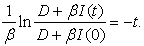Using the boundary condition I(0) = Q, we get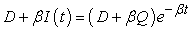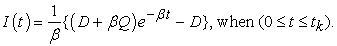(2)

Now for the 2nd period that is during lead time,Therefore from equation (2),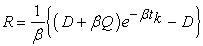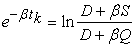(3)

Therefore, mean cycle time,(4)

The holding cost up to reorder point from start period and the holding cost from the start of lead time to the end of a cycle or the start of a new cycle.

Therefore, holding cost(5)

Ordering cost per cycle is the ratio of fixed ordering cost and mean cycle time, so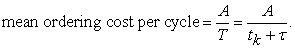Putting the value of tk, we get,(6)

Expected shorted per cycle n(R) can be found by calculating total shortage starting from the beginning of a cycle to the end, so(According to Gel and Keskinocak) 42.

where,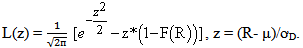Therefore,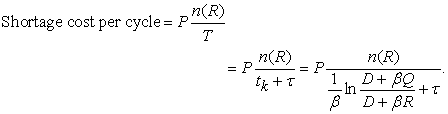(7)

Adding ordering cost, holding cost and shortage cost, we get

Total Cost,Therefore the objective is to minimize,(8)

Differentiating Total cost with respect to Q,(9)

Total cost is differentiated with respect to R,(10)

The second differentials of total cost with respect to Q and R are positive. So it is a minimum point.

Fill-rate, b= 1 - (expected shortage)/order quantity = 1 - n(R)/Q.

### 3. Numerical Example and Analysis

3.1. Numerical Example

The implication of the model is shown below using an example where the values for the parameters are,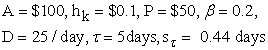• Figure 3. Optimal values of R, Q, Total Cost and shortage

We get, Ropt = 80 units, Qopt = 94 units, TCopt = \$ 4814 and for the optimal values of the variables, fill-rate b = 50%. The graphical representations of the optimal values are shown by Figure 3.

From Figure 3, it is seen that when R=80, Q1 = Q2 = 94. Therefore, Ropt = 80 and Qopt = 94. And the optimal total cost TCopt = \$4814.

3.2. Sensitivity Analysis

A sensitivity analysis has been performed by varying different parameters by 10%-100%. The parameters are:

• Holding Cost, Hk

• Demand elasticity, β

• Shortage Cost, P

• Ordering Cost, A

As a result, optimum values of Q, R, b and total cost responded in certain manners. From these responses the sensitivity of each parameter has been observed. The graphical representations of the sensitivity analysis are as follows.

• Figure 4. Effects of holding cost on reorder quantity
• Figure 5. Effect of holding Cost on quantity ordered
• Figure 6. Effect of holding Cost on total Cost
• Figure 7. Effect of holding Cost on fill-rate
• Figure 8. Effect of demand elasticity on reorder quantity
• Figure 9. Effect of demand elasticity on order quantity
• Figure 10. Effect of demand elasticity on total Cost
• Figure 11. Effect of demand elasticity on fill-rate
• Figure 12. Effect of shortage cost on reorder quantity
• Figure 13. Effect of shortage cost on order quantity Q
• Figure 14. Effect of shortage cost on total cost
• Figure 16. Effect of ordering cost on order quantity
• Figure 17. Effect of ordering cost on total cost
• Figure 18. Effect of ordering cost on fill-rate

Figure 4 & Figure 5 shows inversely proportional relationship of reorder quantity and order quantity with holding cost. Figure 6 shows a proportional relationship between holding cost and total cost, whereas the relationship between holding cost and fill-rate is somewhat pattern-less and cryptic as illustrated by Figure 7. The relationship between demand elasticity is non-commensurate with both reorder quantity and order quantity as depicted in Figure 8 and Figure 9, although the relationship between demand elasticity and order quantity is a weak one compared to the prior one. Figure 10 delineates that total cost reduces almost exponentially with increase in demand elasticity. Figure 11 shows an irregular inversely proportional relationship between demand elasticity and fill-rate. Figure 12 shows that an increase in shortage cost causes reorder point to augment, although the relationship is frail. Increasing shortage cost also causes order quantity to increase as shown in Figure 13. Figure 14 substantiates that, since shortage cost is a constituent of total cost, it exhibits a commensurate relationship to total cost. There is a patent proportionality between ordering cost and reorder quantity demonstrated in Figure 15. Order quantity increases slightly irregularly with increasing fixed order cost depicted in Figure 16. Figure 17 shows that total cost generally goes up with an increasing fixed ordering cost. The relationship between ordering cost and fill-rate does not follow any pattern whatsoever as seen in Figure 18. From the above graphs, it can be concluded that the two most sensitive parameters for this inventory optimization problem are holding cost and demand elasticity. Therefore, it is incumbent upon this type of business to keep a close eye on these two parameters to control inventory cost as a whole.

### 4. Conclusion and Future Work

This model generates a policy to optimize inventory cost. It also illustrates the effect of the order quantity and reorder point on fill-rate. This is a hybrid model that combines deterministic stock-dependent demand with stochastic lead time demand, which is applicable for a wide range of products starting from clothes to food items. Since garment products earn a huge percentage of GDP for Bangladesh, such an inventory optimization model would help RMG flourish more efficiently as most of the garment factories in this country run sub-optimally. This is a much more realistic model compared to previous models concerning stock-depended demand as it takes both demand elasticity and randomness in account. The previous deterministic models assumed demand to be stock-dependent throughout the cycle time and allowed back-logging to avoid shortage cost. But in practice, minimizing shortage is possible only by avoiding stock-outs which is possible by considering uncertainties involved in demand. Furthermore, this article provides a general idea on the impact of the parameters (holding cost, shortage cost etc.) on inventory decisions. This might help business to decide on prioritizing the parameters. The parameter which is most prepossessing should be taken care of in the first place. Unlike previous models of inventory management, this model generates a hybrid approach which has brought focus on lead time demand uncertainty which is the most impactful factor that causes business to hamper.

This work can be expanded to optimize inventory of items for which demand is seasonal and not stock-dependent. Therefore the range of this policy can be broadened using the same modeling approach. This model can be reformulated using other stochastic approach like (s,S) or base-stock model. Such reformulation may assist comparison of inventory models based on separate approaches and decide on which approach would be better in different scenarios. Demand has been assumed to be continuous in this case, which may not the case for most of the products. Therefore this research work provides a potential to formulate inventory models for discrete demand items as well. Apart from using different modeling approaches, such optimization models can be formulated using tools of operations research (OR) such as dynamic programming or integer programming etc. Therefore this research can be reformulated using concepts of OR.

### References

  Dave, U., Patel, L. K. (1981). “(T, Si) policy inventory model for deteriorating items with time proportional demand”, Journal of the Operational Research Society, 32:137-142. In article View Article  Chung, K. J., Ting, P. S. (1993). “A heuristic for replenishment for deteriorating items with a linear trend in demand”, Journal of the Operational Research Society 4:1235-1241. In article View Article  Wee, H. M. (1995). “A deterministic lot-size inventory model for deteriorating items with shortages and a declining market”, Computers & Operations Research - Journal 22:345-356 Publisher Full Text. In article View Article  Chang, H. J., Dye, C. Y. (1999). “An EOQ model for deteriorating items with time varying demand and partial backlogging”, Journal of the Operational Research Society 50:1176-1182. In article View Article  Goyal, S. K., Giri, B. C. (2001). “Recent trends in modeling of deteriorating inventory”, European Journal of Operational Research 134:1-16. In article View Article  Ouyang, W., Cheng, X. (2005). “An inventory model for deteriorating items with exponential declining demand and partial backlogging”, Yugoslav journal of operations research 15(2): 277-288. In article View Article  Alamri, A. A., Balkhi, Z. T. (2007). “The effects of learning and forgetting on the optimal production lot size for deteriorating items with time varying demand and deterioration rates”, International Journal of Production Economics 107: 125-138. In article View Article  Dye, C. Y., Ouyang, L. Y., Hsieh, T. P. (2007). “Deterministic inventory model for deteriorating items with capacity constraint and time-proportional backlogging rate”, European Journal of Operational Research 178(3):789-807. In article View Article  Liao, J. J. (2008). “An EOQ model with non instantaneous receipt and exponential deteriorating item under two-level trade credit”, International Journal of Production Economics 113: 852-861. In article View Article  Lee, W. C., Wu, J. W. (2004). “A Note on EOQ model for items with mixtures of exponential distribution deterioration, Shortages and time-varying demand”, Journal of Quality and Quantity, 38, 457-473. In article View Article  Huang, Y. F., Hsu, K. H. (2008). “A note on a buyer-vendor EOQ model with changeable lead-time in supply chain”, Journal of Information & Optimization Sciences, 29, 305-310. In article View Article  Pareek, S., Mishra, V. K., Rani, S. (2009). “An inventory model for time dependent deteriorating item with salvage value and shortages”, Math Today 25:31-39. In article View Article  Skouri, K., Konstantaras, I., Papachristos, S., Ganas, I. (2009). “Inventory models with ramp type demand rate, partial backlogging and weibull deterioration rate”, European Journal of Operational Research192:79-92. In article View Article  Mishra, V. K., Singh, L. S. (2010). “Deteriorating inventory model with time dependent demand and partial backlogging”, Applied Math Science 4(72):3611-3619. In article View Article  Mandal, B. (2010). “An EOQ inventory model for Weibull distributed deteriorating items under ramp type demand and shortages”, Opsearch 47(2):158-165. In article View Article  Mishra, V. K., Singh, L. S. (2011). “Inventory model for ramp type demand, time dependent deteriorating items with salvage value and shortages”, International Journal of Applied Mathematics and Statistics 23(D11):84-91. In article View Article  Mishra, V. K., Singh, L. S. (2013). “An inventory model for deteriorating items with time-dependent demand and time-varying holding cost under partial backlogging”, Journal of Industrial Engineering International, 9: 4. In article View Article  Trivedi, N. D., Shukla, N. J., Shah, N. H. (2014). “Inventory Model for Deteriorating Items with Fixed Life under Quadratic Demand and Nonlinear Holding Cost”, International Journal of Engineering and Innovative Technology (IJEIT) Volume 3, Issue 12. In article View Article  Gupta, R., Vrat, P. (1986). “Inventory model for stock-dependent consumption rate”, Opsearch, 23 (1), 19-24. In article  Mandal, B. N., Phaujdar, S. (1989). “A note on an inventory model with stock dependent consumption rate”, Opsearch, 26 (1), 43-46. In article View Article  Baker, R. C., Urban, T. L. (1988). “A deterministic inventory system with an inventory-level-dependent demand rate”, Journal of the Operational Research Society, 39 (9), 823-831 In article View Article  Datta, T. K. and Pal, A. K. (1990). “A note on an inventory model with inventory-level dependent demand rate”, Journal of the Operational Research Society, 41 (10), 971-975. In article View Article  Sarker, B. R., Mukherjee, S., Balan, C. V. (1997). “An order level lot-size inventory model with inventory-level-dependent demand and deterioration”, International Journal of Production Economics, 48, 227-236. In article View Article  Chang, C. T. (2004). “Inventory models with stock dependent demand and nonlinear holding costs for deteriorating items”, Asia Pac. J. Oper. Res., 21, 435. In article View Article  Yang, C. T. (2014). “An inventory model with both stock-dependent demand rate and stock-dependent holding cost”, International Journal of Production Economics, Volume 155, Pages 214-221. In article View Article  Du, W., Gao, W., Tang, X. (2015). “A partial backlogging inventory model for non-instantaneous deteriorating items with special stock-dependent demand”, Control and Decision Conference (CCDC), 27th Chinese INSPEC Accession Number: 15341499. In article View Article  Maragatham, M., Palani, R. (2017). “An Inventory Model for Deteriorating Items with Lead Time price Dependent Demand and Shortages”, Advances in Computational Sciences and Technology ISSN 0973-6107 Volume 10, pp. 1839-1847. In article View Article  Montgomery, D. C., Bazarra, M. S., Keswani, A. K. (1973). “Inventory models with a mixture of backorders and lost sales”, Naval Research Logistics Quarterly, 20, 255-265. In article View Article  Rosenberg, D. (1979). “A new analysis of a lot-size model with partial backlogging”, Naval Research Logistics Quarterly, 26 (2), 349-353. In article View Article  Padmanabhan, G., Vart, P. (1995). “EOQ models for perishable items under stock-dependent selling rate”, European Journal of Operational Research, 86, 281-292. In article View Article  Zeng, A. M. (2001). “A partial backordering approach to inventory control”, Production Planning & Control, 12 (7), 660-668. In article View Article  Browne, S., Zipkin, P. (1991). “Inventory models with continuous, stochastic demands”, The Annals of Applied Probability, Vol. 1, No. 3, 419-435. In article View Article  Bashyam, S., Micheal, C. F. (1998). “Optimization of (s, S) Inventory Systems with Random Lead Times and a Service-level Constraint”, Journal of Management Science, Volume 44, Issue-12, Part-2. In article View Article  You, F., Grossmann, I. E. (2008) “Stochastic Inventory Management for Tactical Process Planning under Uncertainties: MINLP Models”, AIChE Journal, 57, 5, 1250-1277. and Algorithms”. In article View Article  Kumar, A., Sharman, G. (1992) “We Love Your Product, But Where Is It?”, Sloan Management Review, Winter, 93-99. In article View Article  Lee, H. L., Billington, C. (1992) “Managing Supply Chain Inventory Pitfalls and Opportunities”, Sloan Management Review, Vol.33, No. 3, pp. 65-73. In article View Article  Johnson, M. E., Lee, H. L., Davis, T., Hall, R. (1995) “Expression For Item Fill-Rates In Periodic Inventory System”, Naval Research Logistics, Vol. 42, pp. 57-80. In article View Article  Lee H. L. (1996). “Effective Inventory and Service Management Through Product and Process Redesign”, Operations Research, pp. 151-159. In article View Article  Voudouris, V. T. (1996). “Mathematical Programming Techniques To De-bottleneck The Supply Chain Of Fine Chemical Industries”, Computers and Chemical Engineering, Vol. 20, pp. 1269-1274. In article View Article  Sabri E. H., Beamon, B.M. (1999) “A Multi-Objective Approach To Simultaneous Strategic And Operational Planning In Supply Chain Design”, The International Journal of Management Science, Omega, Vol. 28, Issue5, pp. 581-598. In article View Article  Teunter R. H., Babai, M.Z., Syntetos, A.A. (2010). “ABC Classification: Service-levels and Inventory Costs”, Production and Operations Management, Vol. 19, No. 3, pp. 343-352. In article View Article  Gel, E., Keskinocak, P. (2013). “Lot size/Reorder level (Q,R) Models”, ISYE 3104. In article

Published with license by Science and Education Publishing, Copyright © 2018 Nasib Al Habib and Shuva GhoshThis work is licensed under a Creative Commons Attribution 4.0 International License. To view a copy of this license, visit http://creativecommons.org/licenses/by/4.0/